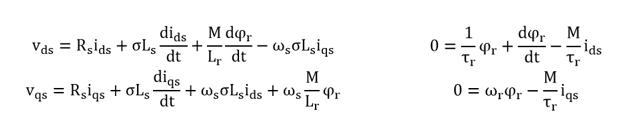# Torque control

EXERCISE: TORQUE CONTROL OF AN INDUCTION MOTOR

An asynchronous machine is controlled in IRFO (Indirect Rotor Field Orientation). It is a current control; the speed loop is therefore non-existent and the user directly imposes the current references. The three-phase d-q diphase transformation used is that of Clarke.Dynamic modeling of the three-phase asynchronous machine permits to establish in d-q reference:With:
Rs, Ls, ωs,  iqs, ids are respectively the resistance, the inductance, the pulsation and the currents of the stator. φr, Lr, ωr are respectively the flux, the inductance and the pulsation of the rotor.

M : Mutual inductance between stator and rotor.

σ = 1 – M2/LsLr           τr=  Lr/R   : Rotor electric time constant            τs =  Ls/Rs   : Stator electric time constant

1- What is this type of control ? On which flux vector the axis “d” of the rotating reference should be directed ?

2- The term M/Lr(dφr/dt) is neglected and the terms  ωsσLsiqs ,  ωsσLsids  ,     ωs(M/Lr)×φare called coupling terms. They can be compensated. Show that the transfer function of the currents of the machine for both axes is:p is the Laplace operator. V’ds and V’qs have to be defined
3- Show that the rotor flux φr responds with a first order time constant τr .Write its transfer function.
4- Find out how to generate the rotor pulsation ωr  and then the Park angle θs.

5- Show that the electromagnetic torque is written : Ce =( 3p/2)×(M/Lr)×φriqs
From this last expression of the torque, explain the principle of vectorial control with Rotor Field Orientation.

CORRECTION